Thursday , July 7 2022# NCERT 5th Class (CBSE) Mathematics: Polygons And Circles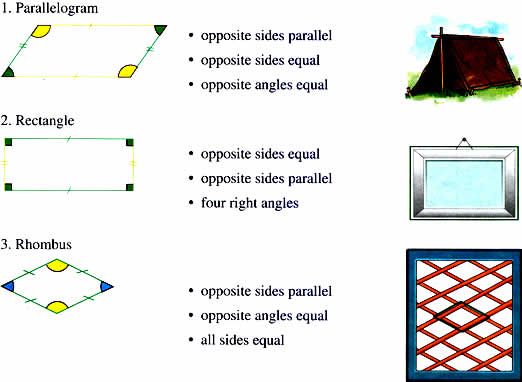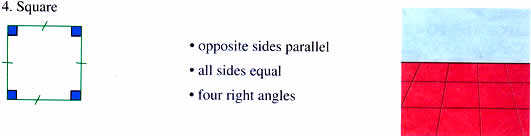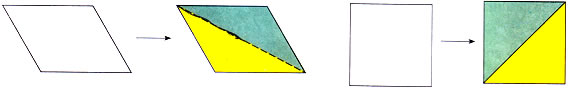Each has a diagonal that divides the quadrilateral into two triangles.

We know that the sum of angles in a triangles is 180°.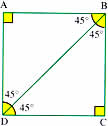Since each quadrilateral has two triangles, the sum of angles in the quadrilateral = 180° × 2 = 30°.

Square ABCD has ∠A = ∠B = ∠C = ∠D = 90°

Sum of the angles in Δ ABD = 180° (90° + 45° + 45°)

Sum of the angles in ΔBCD = 180° (90° + 45° + 45°)

Sum of the angles in ΔABD + Sum of the angles ΔBCD = 360°

Sum of the angles in square ABCD =360°

You can check this out for yourself.

1. Draw and cut out a quadrilateral.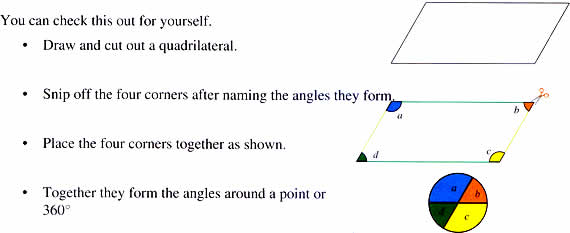If you know the measure of three angles of a quadrilateral, you can find the fourth angle.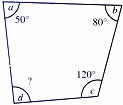∠a + ∠b + ∠c = 50 + 80 + 120 = 250°

∠d = 360 – 250 = 110°

## चुनौती हिमालय की 5th NCERT CBSE Hindi Rimjhim Ch 18

चुनौती हिमालय की 5th Class NCERT CBSE Hindi Book Rimjhim Chapter 18 प्रश्न: लद्दाख जम्मू-कश्मीर राज्य में …